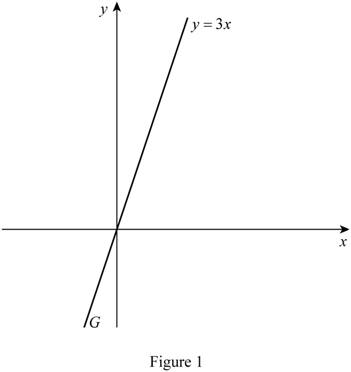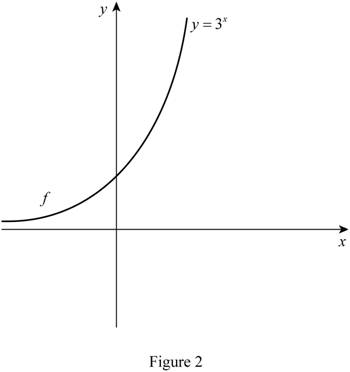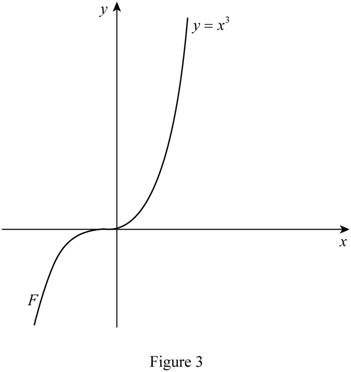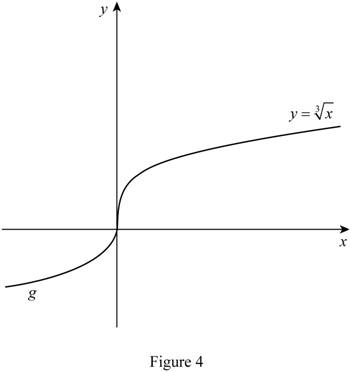# To match: The equation y = 3 x with its graphs without using a computer or graphing calculator.### Single Variable Calculus: Concepts...

4th Edition
James Stewart
Publisher: Cengage Learning
ISBN: 9781337687805### Single Variable Calculus: Concepts...

4th Edition
James Stewart
Publisher: Cengage Learning
ISBN: 9781337687805

#### Solutions

Chapter 1.2, Problem 4E

(a)

To determine

## To match: The equation y=3x with its graphs without using a computer or graphing calculator.

Expert Solution

The equation y=3x matches with the graph G.

### Explanation of Solution

The equation y=3x is in the form of y=mx that represents the equation of straight line.

Here, the slope (m) of the straight line is 3 and the y-intercept is 0.

Thus, the graph with slope m=3 and y-intercept is 0 is shown in Figure 1.From Figure 1, it can be concluded that the graph of the equation y=3x matches with the graph G.

(b)

To determine

### To match: The equation y=3x with its graphs without using a computer or graphing calculator.

Expert Solution

The equation y=3x matches with the graph f.

### Explanation of Solution

The equation y=3x represents an exponential function passing through the point (0,1).

Even for the null value of x, the value of y remains 1, so the graph of the equation y=3x will be on the positive half of y-axis.

Thus, the graph of the equation y=3x is shown in Figure 2.From Figure 2, it can be concluded that the graph of the equation y=3x matches with the graph f.

(c)

To determine

### To match: The equation y=x3 with its graphs without using a computer or graphing calculator.

Expert Solution

The equation y=x3 matches with the graph F.

### Explanation of Solution

The equation y=x3 represents a polynomial function that is cubic in nature.

For the null value of x, the value of y is zero, so the graph of the equation passes through the origin (0,0).

Thus, the graph of a polynomial function with three roots is shown in Figure 3.From Figure 3, it can be concluded that the graph of the equation y=x3 matches with the graph F.

(d)

To determine

### To match: The equation y=x3 with its graphs without using a computer or graphing calculator.

Expert Solution

The equation y=x3 matches with the graph g.

### Explanation of Solution

The equation y=x3 represents a polynomial function with power value of 13.

The graph of a polynomial equation with power (13) is shown in Figure 4.From Figure 4, it can be concluded that the graph of the equation y=x3 matches with the graph g.

### Have a homework question?

Subscribe to bartleby learn! Ask subject matter experts 30 homework questions each month. Plus, you’ll have access to millions of step-by-step textbook answers!